• 最近在分析多个sheet数据发现要求一系列数据平均值,很是麻烦,下面给出VBA的调用代码 在Excel中按下Alt+F11调出VBA编辑器,然后点击插入一个模块,运行代码求平均值 Sub vba计算平均值() Start = 19 For x...
最近在分析多个sheet表的数据发现要求一系列数据的平均值,很是麻烦,下面给出VBA的调用代码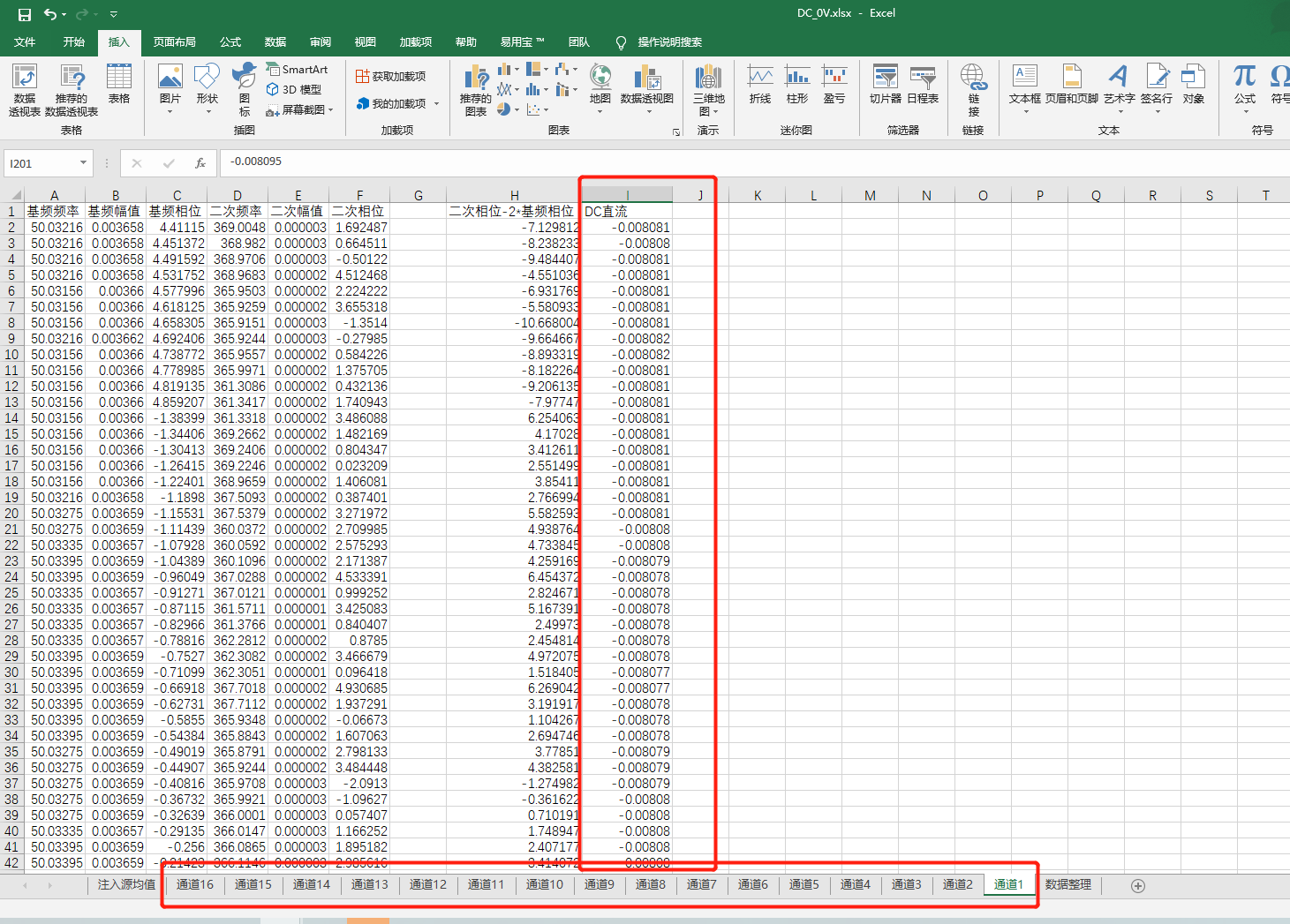在Excel中按下Alt+F11调出VBA编辑器,然后点击插入一个模块,运行代码求平均值


Sub vba计算平均值()

Start = 19
Cells(4, 1) = "平均值"
For x = 2 To 17 Step 1   '根据sheet表的编号来循环
Cells(3, Start - x) = Sheets(x).Name '获取工作表
Sum = 0
num = 0
For j = 2 To 201     '从第二行执行到201行
Sum = Sum + ThisWorkbook.Worksheets(x).Cells(j, 9) '第9列开始的2-201行开始叠加
num = num + 1   '记录计算次数
Next j
Sum = Sum / num    '计算平均值
Cells(4, Start - x) = Sum
Next x
End Sub



最后运行出来的效果如下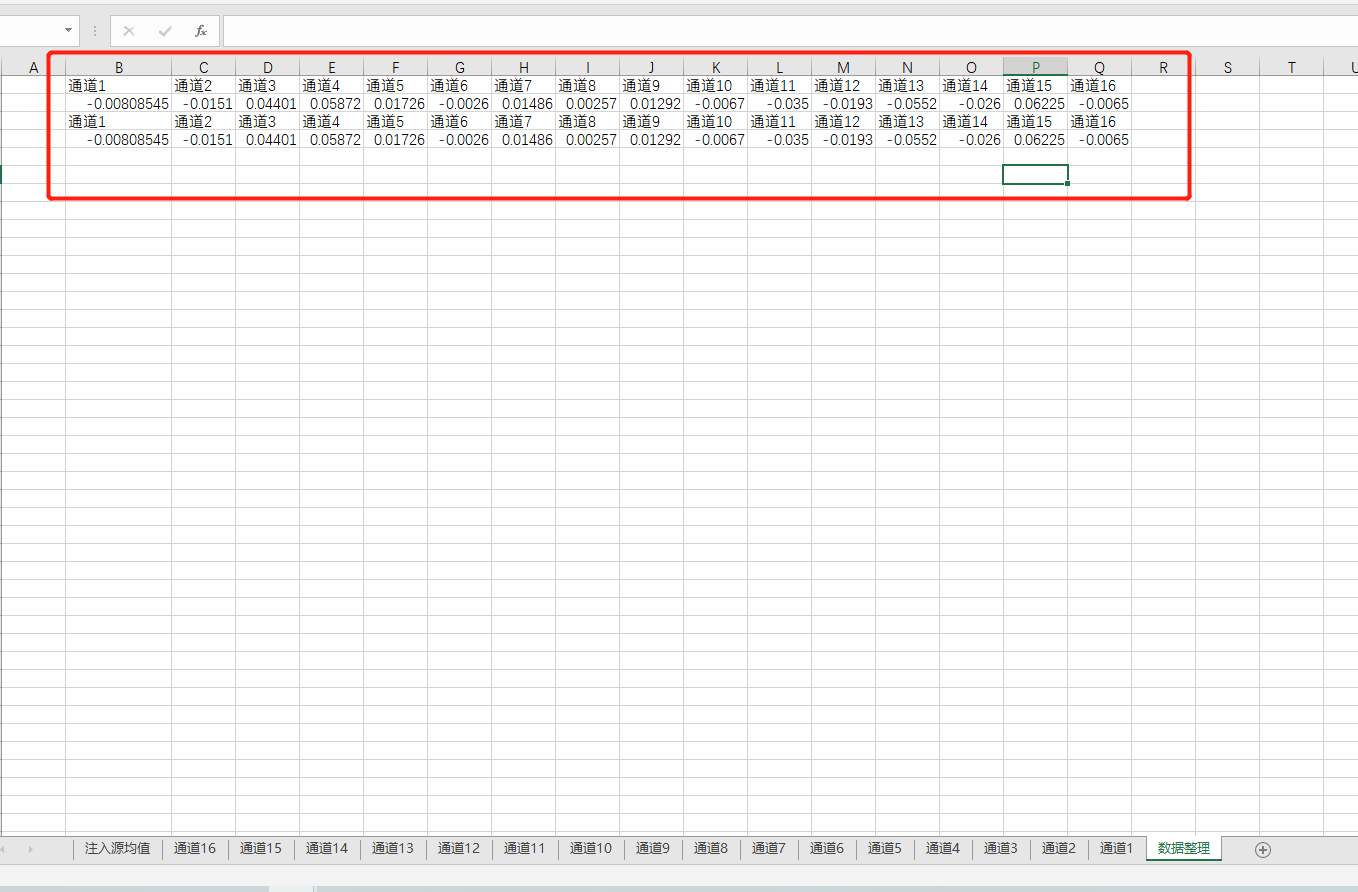展开全文• import os,glob,sys from datetime import date from xlrd import open_workbook,xldate_as_tuple from xlwt import Workbook sys.argv.append('./') sys.argv.append('a.xls') sys.argv.append("b.xls") ...
import  os,glob,sys
from  datetime import date
from  xlrd import open_workbook,xldate_as_tuple
from xlwt import  Workbook
sys.argv.append('./')
sys.argv.append('a.xls')
sys.argv.append("b.xls")
sys.argv.append("b1.xls")
input_floder=sys.argv
output_file=sys.argv
output_workbook=Workbook()
all_data=[]
sales_column_iindex=3
for input_file in glob.glob(os.path.join(input_floder,"*.xls")):
with open_workbook(input_file)as workbook:
list_of_totals=[]
list_of_numbers=[]
workbook_output=[]
for worksheet in workbook.sheets():
total_sales=0
number_of_sales=0
worksheet_list=[]
worksheet_list.append(os.path.basename(input_file))
worksheet_list.append(worksheet.name)
for row_index in range(1,worksheet.nrows):
try:
total_sales+=float(str(worksheet.cell_value(row_index,sales_column_iindex)).strip('$').replace(',','')) number_of_sales+=1 except: total_sales+=0 number_of_sales+=0 print(total_sales,number_of_sales) average_sales='%.2f'%(total_sales / number_of_sales) worksheet_list.append(total_sales) worksheet_list.append(float(average_sales)) list_of_totals.append(total_sales) list_of_numbers.append(float(number_of_sales)) workbook_output.append(worksheet_list) workbook_total=sum(list_of_totals) workbook_average=sum(list_of_totals)/sum(list_of_numbers) for list_element in workbook_output: list_element.append(workbook_total) list_element.append(workbook_average) all_data.extend(workbook_output) for list_index,output_list in enumerate(all_data): for element_index,element in enumerate(output_list): output_worksheet.write(list_index,element_index,element) output_workbook.save(output_file)  附：b.xls b1.xls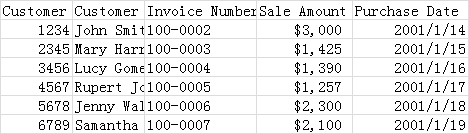展开全文• 知识点如下：pandas的函数、读取多个工作簿下的多个工作表、同时向一个Excel文件内写入多个sheet，一次写入多个Excel文件 源码如下：含主要部分注释： #计算每个工作簿的工作表中值的总和与平均值，写入原文件(这里... 文件路径如下：ExcelPython.py是当前运行文件，红框内三个文件是原始数据文件，每个文件内有三个sheet,含日期类型。如下：我们要计算每个工作簿的工作表中值的总和与平均值，写入原文件(这里先复制出一份和原来一样的文件后写入新文件) 知识点如下：pandas的函数、读取多个工作簿下的多个工作表、同时向一个Excel文件内写入多个sheet，一次写入多个Excel文件 源码如下：含主要部分注释：#计算每个工作簿的工作表中值的总和与平均值，写入原文件(这里先复制出一份和原来一样的文件后写入新文件) def read_sum_excel_2(): all_workbooks = glob.glob(os.path.join(os.getcwd(),'sales_*.xlsx')) for workbook in all_workbooks: output_file = 'excel_python_write_{}'.format(os.path.basename(workbook).split('sales_')) print(output_file) all_worksheets = pd.read_excel(workbook,sheet_name=None) writer = pd.ExcelWriter(output_file) workbook_total_sales = []#每个excel文件内所有sheet加起来的total_sales总数 workbook_total_averages = []#每个excel文件内所有sheet加起来的total_averages平均数 for worksheet_name,data in all_worksheets.items(): #处理日期格式 data['Purchase Date'] =data['Purchase Date'].dt.strftime('%Y-%m-%d') #计算总Sale Amount workbook_total_sales.append(data['Sale Amount'].sum()) total_sale_frame = pd.DataFrame(['Sale_Amount_total','','',data['Sale Amount'].sum(),'']).T total_sale_frame.columns = data.columns df_new = pd.concat([data,total_sale_frame],ignore_index=True) #计算平均Sale Amount workbook_total_averages.append(data['Sale Amount'].mean()) average_sale_frame = pd.DataFrame(['Sale_Amount_average','','',data['Sale Amount'].mean(),'']).T average_sale_frame.columns = df_new.columns df_new = pd.concat([df_new,average_sale_frame],ignore_index=True) #一个excel写入多个sheet df_new.to_excel(writer,sheet_name=worksheet_name,index=False) #每个工作簿下工作表信息 workbook_total = pd.DataFrame(workbook_total_sales).sum() workbook_average = pd.DataFrame(workbook_total_averages).mean() workbook_stats_data = { 'workbook_total':workbook_total, 'workbook_average':workbook_average} #每个工作簿下工作表信息总和 workbook_stats_frame = pd.DataFrame(workbook_stats_data,columns=['workbook_total','workbook_average']) workbook_stats_frame.to_excel(writer,sheet_name='count',index=False)#将总和写入新的sheet里，作为全表统计信息 print(workbook_stats_frame) writer.save()  运行，结果如下：原表信息拷贝过来，日期类型做了处理，且新增了两行和一个sheet，新增count sheet显示如下：展开全文python excel • 往往我们要手工合并，在进行求和、将多个单元格信息合并到一个单元格、求平均值、求排名 等等的处理。 而现在有一款方便的工具，可以一键汇总收集的信息（信息要按行）。 工具地址：www.excelfb.com 点击：...  作为收集信息的人员，往往面临要把收集来的信息进行汇总的情况。往往我们要手工合并，在进行求和、将多个单元格信息合并到一个单元格、求平均值、求排名 等等的处理。 如何按照某几列相同的值，或Sheet名、文件名，条件汇总信息？ 而现在有一款方便的工具，可以一键汇总收集的信息（信息要按行）。 工具地址：www.excelfb.com 点击：汇总工具---》Excel自动汇总工具（根据汉字描述的要求格式进行汇总） 如图所示，2018年与2019年的信息是两个文件，现在要对两个文件中的内容进行汇总。操作如下图：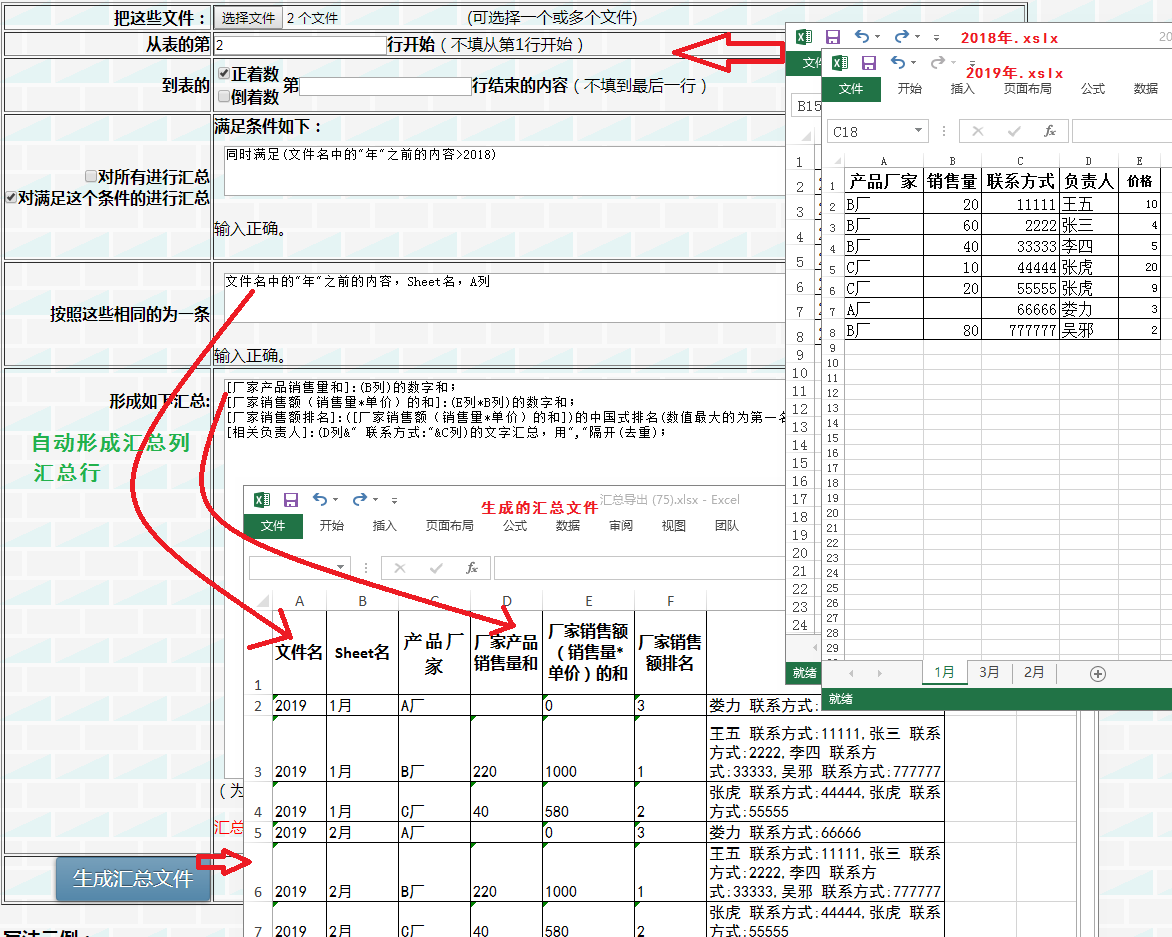如果，只是想把信息合并到一个表，可以使用 合并工具 ，如下图所示：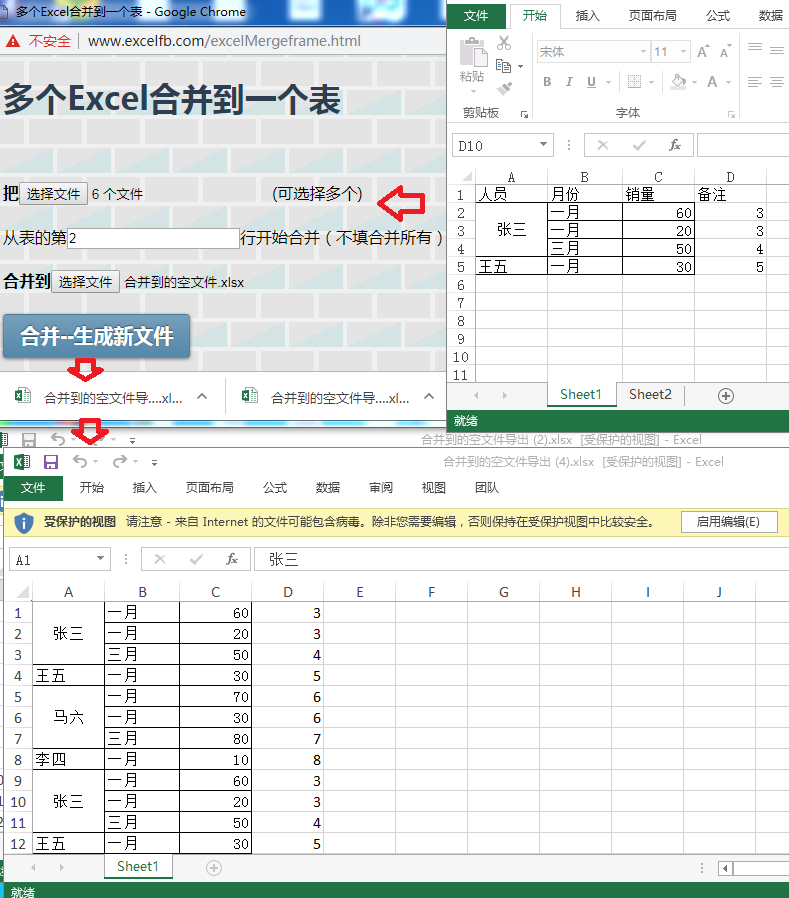如果想提取信息，使用 从多个Excel文件中查东西（提取到新表），如下图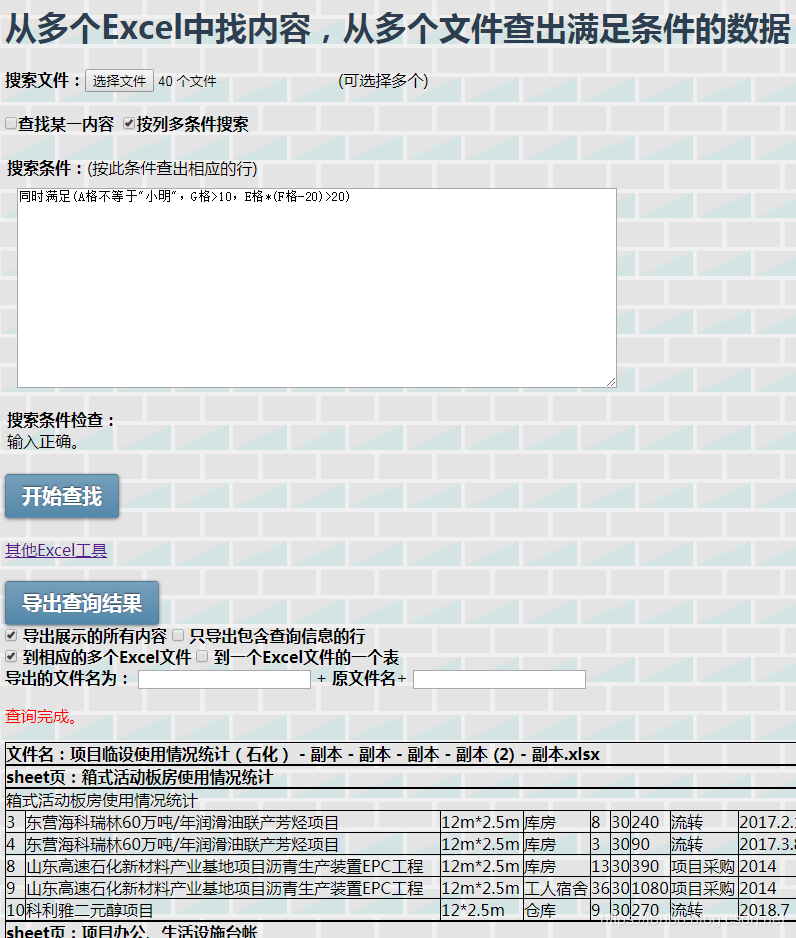展开全文• 做为信息收集人员，可能经常需要统计多个Excel文件收集来的信息，对多个Excel、对多个工作簿、对多个表格进行统计，统计的维度包含按文件名、按表名进行求和、求排名、求平均值、条件统计、条件求和、文字汇总、多... • Excel单个单元格多个数值求平均值 标签： Excel办公求和实用单元格 办公软件相关（3） 下面例子以office2007为例操作 数据格式如下图： EVALUATE(A1) 这个是核心 1：首先求和 ... • 学习了Python相关数据类型，函数的知识后，利用字符串的分割实现了输入任意多个数据，并计算其平均值的小程序。思路是接收输入的字符串，以空格为分隔符，将分割的数据存入列表（lst1）中，将lst1中的数据转存入另一... • 选择单元格的数字区域，只显示计数，而没有合计和平均值。 并且单元格格式已经设置了数值格式。 原因： 该问题由于虽然设置了该列的单元格为数值格式，但是单元格还没有转换为数值格式。具体你可以双击下每...Excel • 【技术关键】1、从excel把数据读到数据框2、算法实现将数据框的一些数据合为新的数据并组成新的数据框3、将处理结果，即新的数据框保存到excel文件（或.csv）4、将绘图结果输出到PDF文件保存最近在尝试分析近日的...R语言 • 1.用到的函数 M=csvread（‘filename.csv’,R,C）. 从第R行、第C列开始，将filename.csv文件中的数据（只能是数字）写入矩阵M中。...若当前路径中不存在filename.csv这文件，会自动生成改文件，这一点...Matlab 文件操作 CSV • excel的一列有n数，我想让这n数的每100数取平均值，放在后面一列，比如说B1=A1到A100的平均值，B2=A101到A200的平均值 在B1单元格输入以下公式，然后向下填充公式 =AVERAGE(OFFSET(A$1,(ROW(A1)-1)*100,100,))...excel操作
• EXCEL 多条件求最大值、最小值、平均值、标准偏差 当表格内的数据需要按多个条件进行筛选后求最大值、最小值、平均值、标准偏差时怎么办？同事的这个问题差点把我难倒。经过一番摸索，为便于公式的理解，我将参与...
• 1.从文件中打开数据集、复制粘贴也行，并删除多余的空行 删除不符合样式的数据行 2.调整相关的数据表格列变量属性为数字 调整数据集属性 ...3.将数据依据指定列，按范围...4.按cjdj分组统计成绩的平均值 设置...大数据
• Excel 2007中若要汇总和报告多个单独工作表的结果，可以将每个单独工作表中的数据合并计算到一个主工作表中。这些工作表可以与主工作表在同一个工作簿中，也可以位于其他工作簿中。对数据进行合并计算就是组合数据，...excel microsoft 数据库 工具
• 圣人曾说过：数据和特征决定了机器学习的上限，而模型和算法只是逼近这上限而已。 再好的模型，如果没有好的数据和特征质量，那训练出来的效果也不会有所提高。数据质量对于数据分析而言是至关重要的，有时候它的...python 特征工程
• 如何求特定单元格的平均值 求助EXCEL达人，EXCEL计算范围：A列里程项目大于等于13公里小于15公里，B列自动计算平均值？ 求特定单元格平均值.rar(1.97 KB, 下载次数: 18)   2楼 发表于 2012-4-9 16:05...
• 本篇主要分析结构体数组和结构体类型的函数，对于二级C语言来说，主要是考处理学生多个成绩上和求平均值上。两个实例的参考代码里面都有相应的注释，就不在外面分析了 一、结构体变量 C语言结构体（Struct）从本质...指针 c语言 程序人生
• 在微软POWER BI中的默认聚合运算有以下几种,但是在实际应用中会经常碰见加权平均值的情况,由于加权平均值需要两列数据才能算出,不能使用正常的聚合运算得到,只好采取其他的方式完成。以下就是在POWERBI中实现加权...
• 数据的准备往往占到整个工作的70%的时间。数据准备包括了数据的抽取，清洗，转换，...优点：简单易行，在数据含有多个属性缺失、被删除的含缺失数据与信息中的数据量相比非常小的情况下是非常有效的 缺点：...机器学习
• 1. 单变量数据中检测异常点的方法：绝对中位差：如果一点大于或小于3倍的绝对中位...平均值：对于高斯分布的数据来说，68.27%的数据集中在一标准差的范围内，95.45%在两标准差的范围内，99.73%在3标准差的范...
• 使用工具：Excel插件方方格子 下载地址：http://www.ffcell.com/ 操作如下：
• 在日常的数据分析工作中，数据中的缺失是最头疼的一内容。 在日常数据采集的过程中，由于各种原因在数据采集的过程中会造成数据的部分缺失，一般会用空格、Nan、占位符表示。但是对于应用的机器学习或者深度学习...python 机器学习
• 本章主要内容：创建数据透视表，更改数据透视表汇总方式，数据透视表中的组合，汇总数据，创建计算字段，生成工作表excel
• ## 表格化数据挖掘

万次阅读 多人点赞 2020-12-02 08:39:13
绝大多数的数据都是表格数据（或可以转化为表格数据） 非结构化数据主要分为：图片、声音、视频、文本 在NLP任务中，在情感分类中最早使用字典匹配的方法，如是否包含good这词，通过该方法就将非结构化数据转换成...
• 数据挖掘：数据预处理——缺失处理 一、什么是缺失 缺失是指粗糙数据中由于缺少信息而造成的数据的聚类、分组、删失或截断。它指的是现有数据集中某个或某些属性的是不完全的。 而在数据处理的过程中，缺失...机器学习 python
• 为了方便使用，博文中附上了包括数据集整理及数据预处理在内的所有代码及处理好的数据集，同时对代码进行了解释，其要点如下：UCI数据集介绍、不同数据集的整理程序、148整理好的数据集与对应程序。机器学习 人工智能 MATLAB
• 这其实是应该算是我们Matlab上开辟的第二部分了，第一部分就是之前的Matlab的基础...这是这系列的第一篇，主要介绍最值、平均值和中值。 1.最值 已知一行数字，需要知道数字里面的最大值和最小值，这是...
• 根据以下帖子整理： https://www.cnblogs.com/tiandi/p/7648417.html  https://blog.csdn.net/lijie45655/article/details/49132437  ... 1、准备全国省级行政区数据，需要有省级...
• # -*-coding: utf-8 -*- import pandas as pd ...#求‘ave_time’的平均值 aveTime=df['ave_time'].mean() #把ave_time这列的缺失值进进行填充，填充的方法是按这一列的平均值进行填充 df2=df.fillna(aveTime) #pandas...